# Generating a Tessellation from Statistical Cell Properties

Statistical morphological properties for the cells can be specified as input, using option `-morpho`. In this case, instead of a Voronoi tessellation, Neper generates a Laguerre tessellation (which can represent any tessellation comprised of convex cells, and therefore does not involve particular limitations) by optimizing the attributes of its seeds until the specified input is reached [CMAME2018].

Note

To reproduce exactly the images below, add the following line to your `\$HOME/.neperrc` file (or to a local configuration file to be loaded with `--rcfile`):

```neper -V -imagesize 800:400
```

## Using the Built-in `graingrowth`

Option `-morpho` includes the `graingrowth` (or `gg`, for short) properties, which corresponds to those of an experimental polycrystal subjected to grain growth. Both the cell size distribution and the cell sphericity distribution (how close are the cells from a sphere) are defined [CMAME2018].

A 100-cell tessellation with grain-growth properties can be generated using the Tessellation Module (-T) as follows:

```\$ neper -T -n 100 -morpho gg
```

This produces a Tessellation File (.tess) named `n100-id1.tess`.

The tessellation can be visualized using the Visualization Module (-V):

```\$ neper -V n100-id1.tess -print img1
```

This produces a PNG file named `img1.png`.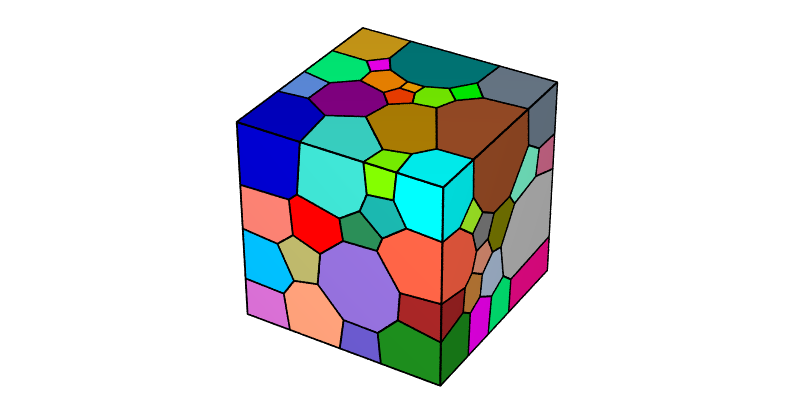## Specifying Custom Properties

Statistical properties other than those of `gg` can be specified. `gg` actually is an alias for `diameq:lognormal(1,0.35),1-sphericity:lognormal(0.145,0.03)`, where `diameq::lognormal(1,0.35)` specifies a lognormal distribution of the (normalized) cell equivalent diameters of average 1 and standard deviation 0.35, and `1-sphericity:lognormal(0.145,0.03)` corresponds to a lognormal distribution of the cell “1-sphericities” of average 0.145 and standard deviation 0.03. It is therefore possible to use this longer argument and change the numerical values. For example, to get nearly equal-size cells (small standard deviation of the cell size lognormal distribution), one may use:

```\$ neper -T -n 100 -morpho "diameq:lognormal(1,0.05),1-sphericity:lognormal(0.145,0.03)"
```

The tessellation can be visualized as before:

```\$ neper -V n100-id1.tess -print img2
```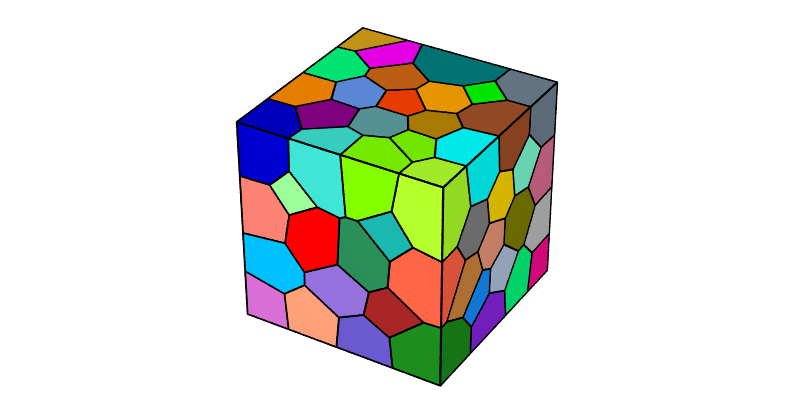Of course, it is also possible to change the sphericity distribution, for instance to get less spherical grains (smaller average of the sphericity distribution):

```\$ neper -T -n 100 -morpho "diameq:lognormal(1,0.05),1-sphericity:lognormal(0.245,0.03)"
```

The tessellation can be visualized as before:

```\$ neper -V n100-id1.tess -print img3
```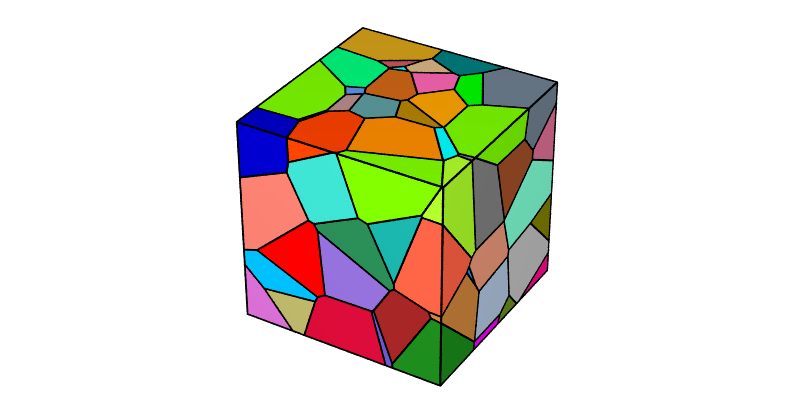Note

See Statistical Distributions for a list of possible distributions and other possible inputs.

Important

Although it is not strictly needed, distributions for both the sizes (e.g. `diameq`) and the shapes of the cells should be specified. Generating a tessellation from only a cell size distribution can lead to unrealistically small cell sphericities.

## Specifying an Absolute Cell Size Distribution

It is possible to get Neper to consider the cell size distribution as a distribution of absolute values by using `-n` `from_morpho` (instead of an actual number of cells). Neper determines the number of cells from the cell size distribution and the domain size. An example is:

```\$ neper -T -n from_morpho -morpho "diameq:lognormal(0.2,0.06),1-sphericity:lognormal(0.145,0.03)" -o mytess
```

Note

Option `-o` is used to set the output file name. The default file name is `n<n>-id<id>.tess` and, in this case, would be the odd `nfrom_morpho-id1.tess`.

The tessellation contains 184 cells and can be visualized as before:

```\$ neper -V mytess.tess -print img4
```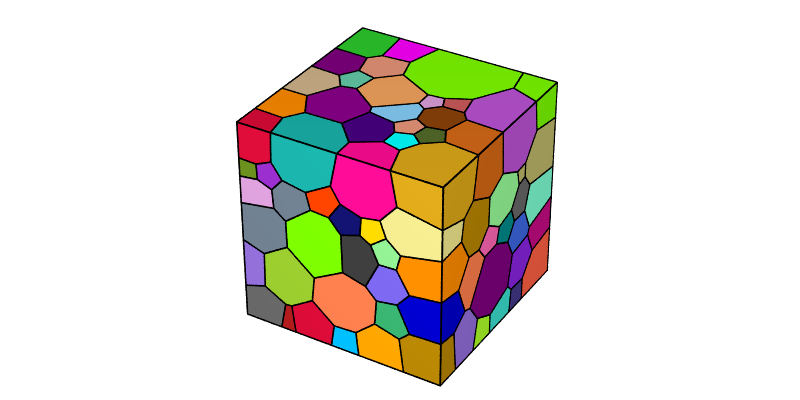## Specifying an Experimental Cell Size Distribution

Experimental or, more generally, numerical distributions defined from a file can be specified by using option `-morpho` `<property>:custom(<file_name>)` (instead of analytical distributions).

Consider a cell-size (`diameq`) distribution defined from a file, `mydistrib`, that contains:

```0.2 10.0
0.3 20.0
0.4  5.0
```

i.e., 10 cells have a `diameq` equal to 0.2, 20 cells have a `diameq` equal to 0.3, and 5 cells have a `diameq` equal to 0.4 (for a tessellation comprising 35 cells). The numbers on the right column are actually only considered relative to each other.

Note

• In contrast to analytical distributions, numerical distributions do not need to be normalized to 1.

• As for an analytical distribution, if `-morpho` `from_morpho` is used, Neper determines the number of cells from the cell size distribution and the domain size.

The corresponding tessellation can be generated as follows:

```\$ neper -T -n from_morpho                                                        \
-morpho "diameq:custom(mydistrib),1-sphericity:lognormal(0.145,0.03)" \
-statcell diameq                                                      \
-o mytess
```

where option `-statcell` is used to output the cell equivalent diameters to file `mytess.stcell`.

The tessellation can be visualized by coloring the cells from their equivalent diameters using options `-datacellcol` and `-datacellscale`:

```\$ neper -V mytess.tess                                       \
-datacellcol "real:file(mytess.stcell)"           \
-datacellscale 0.15:0.20:0.25:0.30:0.35:0.40:0.45 \
-datacellscaletitle "Cell size (mm)"              \
-print img5
```

This produces a PNG file named `img5.png` for the tessellation and a PNG file named `img5-scale3.png` for the scale bar.

To include the scale bar to the image, ImageMagick can be used:

```\$ convert img5.png img5-scale3.png -gravity East -composite img5.png
```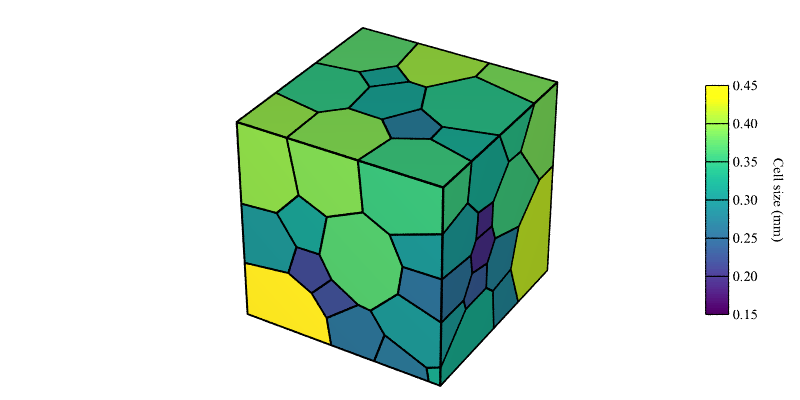## Speeding up Tessellation

When statistical properties (or other properties) are specified as input, Neper employs an interative approach, and so tessellation generation is longer than for a standard Voronoi tessellation. Even though the tessellation generation remains relatively fast, to speed up the tessellation process, a first way is through multithreading, as a performace increase is expected up about 16 threads. A second way is to modify the termination criterion, which is controlled by option `-morphooptistop` and set to `val=1e-5` by default, which is relatively low. The value can be increased, or another criterion can be used.

CMAME2018(1,2)

R Quey and L Renversade, Optimal polyhedral description of 3D polycrystals: method and application to statistical and synchrotron X-ray diffraction data, Computer Methods in Applied Mechanics and Engineering, vol. 330, pp. 308-333, 2018.